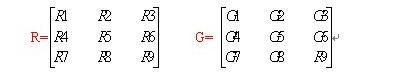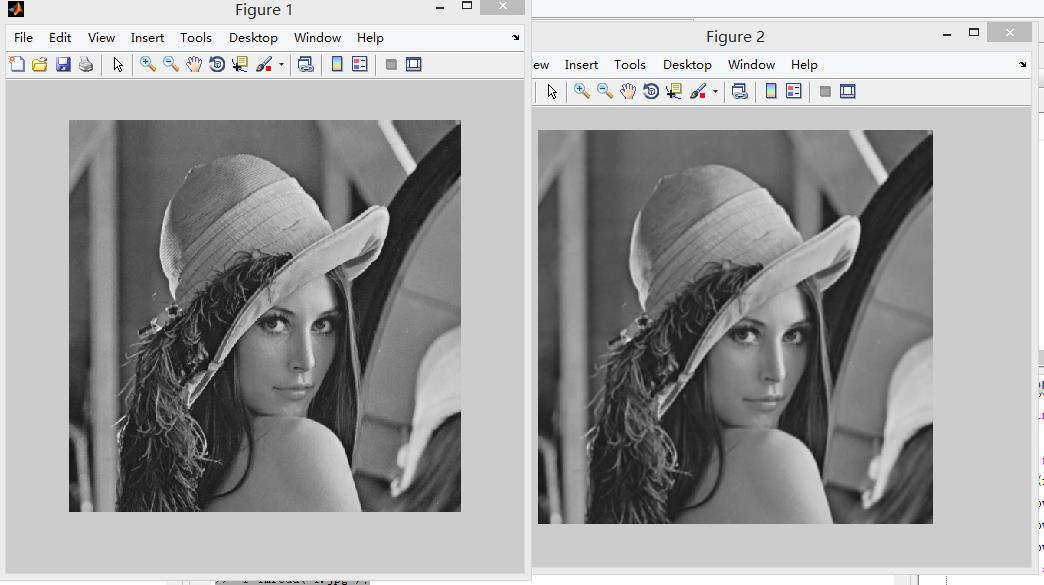# MATLAB数字图像处理（3）滤波

## 线性空间滤波R5(中心像素)=R1G9 + R2G8 + R3G7 + R4G6+ R5G5 + R6G4 + R7G3 + R8G2 + R9G1

MATLAB中提供了函数imfilter()进行滤波操作。

g=imfilter(f,w,'replicate')

f是待处理的图像，w是滤波器，replicate指定通过复制图像边界外的值来扩展。MATLAB提供了函数fspecial()，生成各类二维线形滤波器，函数如下。

w=fspecial('type'parameters)

type滤波器的类型，parameters，可选参数。

  f=imread('1.jpg');
imshow(f)，title('原图');
w=fspecial('average');%3x3(默认)均匀滤波器
f1=imfilter(f,w,'replicate');
figure,imshow(f1)，title('线性滤波直方图');## 非线性空间滤波

f=imread('1.jpg');
f=imnoise(f,'salt & pepper',0.2);
g=medfilt2(f);
g=medfilt2(f,'symmetric');
subplot(1,2,1);imshow(f);title('噪声污染');
subplot(1,2,2);imshow(g);title('中值滤波');©️2019 CSDN 皮肤主题: 大白 设计师: CSDN官方博客